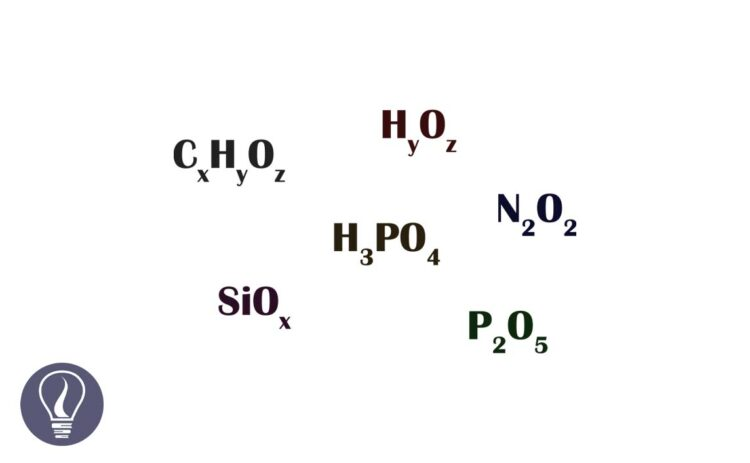More results...

Generic selectors
Exact matches only
Search in title
Search in content
Post Type Selectors

# Empirical Formula vs Molecular FormulaB.Sc (Hons, USJ) (Polymer Science and Technology, Chemistry, Physics)
Categories
Last Updated On : January 20, 2023
Published Date : December 14, 2022

The simplest ratio between the number of mols of atoms in one mole of a compound is known as the empirical formula. In other words, the empirical formula is the simplest ratio between the number of atoms in one molecule. The empirical formula gives an idea about the percentage of each element in a compound.

The formula that shows the exact number of moles of atoms contained in one mole of a compound is called the molecular formula. Or molecular formula shows the exact number of each atom in a molecule.

In a glucose molecule, the number ratio between C: H: O is 1:2:1. Therefore, the empirical formula of glucose is as follows.

The molecular formula shows the exact number of atoms in a molecule whereas the empirical formula shows the simplest ratio between the atoms. But for some compounds, both the empirical formula and molecular formula are the same. The relation between the empirical formula and molecular formula can be expressed as follows.

"n" is always a whole number. (n = 1,2,3,…)

There can be several molecular formulas for one empirical formula. As an example, CH2 empirical formulas have different molecular formulas like C2H4, C3H6, C4H8, etc.

If there are several molecular formulas for one empirical formula, the mass percentages of elements in each formula are the same. Therefore, the Carbon percentage and Hydrogen percentage of above C2H4, C3H6, and C4H8 are the same. Therefore, when we know the mass percentage of a particular compound, both the empirical formula and molecular formula can be derived.

## Derivation of the empirical formula and the molecular formula of a compound

Simply, the empirical and molecular formulas give the mole ratio between atoms in a compound. When the mass percentages of each element in a compound are known, they should be converted into mole ratios to derive the empirical formula.

### Example 01:

The question:

An inorganic compound contains only Sodium (Na), Sulfur (S), and Oxygen (O). Mass percentages of those elements are, Na – 29.11%, S - 40.50%, and O - 30.39%. the relative molar mass of the compound is 157.78 u. Relative atomic masses of Na, S, and O are 22.98 u, 32.06 u, and 15.99 u respectively. Find the empirical and molecular formula of the above compound.

• Mass percentages of each element are taken as the mass ratio.

• To get the mols in the compound, the masses of each element are divided by their relative mass units.

• The above mole ratio is divided by the smallest value to get a whole number.

• After dividing by the smallest number, we still have decimal values in ratio. If there are 0.5 or 0.6 in the first decimal place, all values are multiplied by 2 and rounded to the nearest whole number.
• If there are 0.1, 0.2, 0.8, or 0.9 in the first decimal place, they are directly rounded to the nearest whole number.
• If there are 0.3 or 0.7, all the values are multiplied by 3 and rounded to the nearest whole number.
• Here, all the numbers are multiplied by 2.

• Finally, we get a 2:2:3 ratio for Na: S: O.
• Therefore, the empirical formula of the above compound can be written as Na2S2O3.
• Now we have to find the molecular formula of the above compound.

### Example 02:

The question:

x.H2O is a white color crystalline salt. X contains 19.4% of carbon, 6.4% of hydrogen, 22.6% of nitrogen, and 51.6% of oxygen. Derive the empirical formula of x. (Relative atomic masses; C - 12.01 u, H - 1.00 u, N - 14.00 u, O - 15.99 u)

If the above compound releases 2 mols of Ammonia gas (NH4) out of 1mol of the compound when it is heated find the molecular formula of the compound. Consider NH3 is the only product released from compound x, that contains nitrogen (N).

• Empirical formula – CH4NO2

1 mole of compound release two mols of NH4 means that there are two mols of Nitrogen molecules in one mol of the compound. The empirical formula contains only one mole of nitrogen. Therefore, the empirical formula should be multiplied by 2 to get the molecular formula.